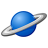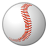# PSoC™ 5, 3 & 1 Forum DiscussionsAnonymous
Not applicable

# Multiple Waveform Generation at the Same Time

Hi everyone, I am working on a project that needs to generate multiple sine waves at the same time to drive a tweeter. The background is that I would like to convert an image into sound waves. Each pixel is going to be designed a specific sine wave with its specific amplitude and frequency.If I would like to convert an image in 64x64 resolution, the amount of sine waves I need to generate is 4096.

Here comes the question, I have looked up a technical document about waveform generation by using WaveDAC8 but the closet example project was actually adding two different sine waves together by using two DAC in parallel. I know its impossible to add thousands of sine waves by using thousands of DACs in PSoC 5 LP. Thus I looked into the limitation of WaveDAC and the result was that I could generate 4 waves at maximum at the same time. An application engineer suggested me to program an algorithm to add those thousands of sine waves together before feeding them into a DMA,then use the DMA channel to transfer the final result to a WaveDAC8.Is this feasible?

If it is feasible, can anyone help me further or share me related technical documents? I have no idea how to add those sine waves before feeding them into a DMA in coding and how can I set the WaveDAC by then. What I can do so far is creating a table of amplitudes and a table of frequencies that corresponding to image pixels through MATLAB coding.

Many thanks!

Lingo

5 RepliesLevel 8
Level 8# Re: Multiple Waveform Generation at the Same Time

It does not really help when you post the same question to multiple, unrelateds forums (your question is neither a "known problem" nor is it related to "device programming").

Answer should be handled here: http://www.cypress.com/forum/psoc-5-architecture/questions-waveform-generation-psoc-5-lpLevel 5
Level 5# Re: Multiple Waveform Generation at the Same Time

The algorithm for generating an array that outputs a sine wave is simple. You can do it in a spreadsheet with the formula =SIN(2*PI()*A1/61)*0.75 where 61 represents the number of samples in a single wave cycle and 0.75 represents amplitude.
If you do many of these in C I think you will soon see certain problems. First, you cannot have 4096 arrays without running out of memory so you would want to re-use arrays after summing the values of two of them.
Second, let's say your array lengths are 128 samples. For any wavelengths that do not have integer multiples of cycles in 128 will not cross at zero on sample  when the output wraps back to sample .  Thus you will be getting severe crossover distortion on those frequencies.  Below is a snippet of code that pre-generates two sine waves of different frequencies and amplitude and sums the two sine waves.  I'm not saying it's a solution;  indeed, I think it shows what problems you might have.  You might want to look for analog solutions in hardware.

#define PI 3.14159265
#define AMPLITUDE1 1
#define AMPLITUDE2 0.5

uint8 i,j;
float sinwave1, sinwave2;
float sumofwaves;
for (i=0; i < 128; i++) sumofwaves = 0.0;

for(i = 0; i < 128; i++)
{
sinwave1 = (i*2*PI/128) * AMPLITUDE1; // End point wraps at zero to next waveform
sinwave2 = (i*2*PI/61) * AMPLITUDE2 ; // Notice that the ends will NOT cross at zero
sumofwaves += sinwave1 + sinwave2;
}
ouput (sumofwaves); // Some function that will output your signalAnonymous
Not applicable

# Re: Multiple Waveform Generation at the Same Time

I was trying to generate a single sine wave, but I failed. The result showed me a 4V dc voltage(Possibly because I set the DAC scale: 0-4.08v) Could you please have a look at my code to see if there is something wrong? I also tried your equation sinewave = (i*2*Pi/800)*Amplitude; (I assumed I need 800 samples per period) , but the results were the same. Maybe I thought was totally wrong? I spent lots time in debugging but couldn't figure it out.

My code:

#include <project.h>
#include <math.h>

#define TABLE_SIZE 1000//amount of samples
#define TWO_PI (3.14159 * 2)
#define Amplitude  1
#define MaxFreq  400
#define Freq_1 200
#define SamplePerPeriod 800//Based on the maximum frequency

int main()
{
float Samples [TABLE_SIZE];
float TimeIncrement = (1/MaxFreq)/SamplePerPeriod;
int DigitalResults [TABLE_SIZE];
float t[TABLE_SIZE];//index of each sampling time point
int i;

VDAC8_1_Start();

for (i = 0; i < TABLE_SIZE; i++)
{
t = i*TimeIncrement;
Samples = Amplitude*sin(TWO_PI*Freq_1*t );

//Samples = ((i*TWO_PI)/SamplePerPeriod)*Amplitude;

VDAC8_1_SetValue(DigitalResults);

CyDelayUs(1); //the time interval is 3.13us
}
// }
return 0;
}

Many thanks!!

Regards,

LingoLevel 10
Level 10# Re: Multiple Waveform Generation at the Same Time

#define PI 3.14159265

We learnt the trick

#define PI   (4*atan(1.0))   // this is a constant evaluated at compile-time with best precision

BobLevel 8
Level 8# Re: Multiple Waveform Generation at the Same Time

You could try to create the tables with the data in a first loop, and then use a second loop to send them to the DAC. That way you can put as breakpoint after the first loop (or send it via UART to the PC) and check that the values in there are indeed correct.